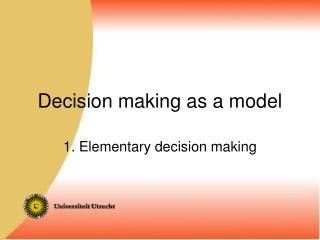DownloadDownload PresentationDecision making as a model

# Decision making as a model

Télécharger la présentation## Decision making as a model

- - - - - - - - - - - - - - - - - - - - - - - - - - - E N D - - - - - - - - - - - - - - - - - - - - - - - - - - -
##### Presentation Transcript

1. Decision making as a model 1. Elementary decision making

2. http://www.fss.uu.nl/psn/web/onderwijs/Edu_Kunst/ Zie onder: Beslissen als Model.

3. Recap: What is a sensible decision? Classical criterion in uncertain situations (e.g. gambling): choose alternative with max expected value (E V) Cf. Pascals Wager (probability theory stems from gambling and theology)

4. Christiaan Huygens stated the principle in 1657: By exempel. So yemandt sonder mijn weeten in d’eene handt 3 schellingen verbergt en in d'ander 7 schellingen ende my te kiesen geeft welck van beyde ick begeere te hebben ick segge dit my even veel weerdt te zijn als of ick 5 schellingen seecker hadde. EV(A) = (p(Au)V(Au)) ` u

5. Heaven Trouble Hell Fun p 1-p Believing Not believing p 1-p Pascals Wager in modern terms: probability that God exists = p p•() + (1-p)•TB  p•(- ) + (1-p)•FNb -  EV(A) = (p(Au)V(Au)) u So: even with small p believing is reasonable!

6. Value, even monetary value, is problematic! N. Bernouilli: Petersburg paradox Payment dependent on first heads: Heads on 1st throw: €2, Heads on 2nd throw: €4 etc……. EV = 1/2•2 + 1/4• 4 + 1/8•8 ………..  D. Bernouilli (1738): subjective utility log-function of monetary value (cf Fechner!) EU(A) = (p(Au)U(Au)) Expected u Utility

7. EU(A) = (p(Au)U(Au)) u But what is utility? Derive from preferences (e.g. with p(A) = 1) Scale properties, Consistency e.g.: if A > B then A&C > B&C Utility function of multiple atributes (MAUT)

8. pz To operate 1-pz pz Not to operate 1-pz EV(A) = (p(Au)V(Au)) u What is probability? Do I know those p(Au)’s ? Sometimes I know B (e.g. test result) and p(B|Au) (statistical data) • Approaches: • Bayes • Neyman-Pearson, SDT

9. Recap Bayes’ Rule Pierre Simon Laplace

10. If some clinical test detects .99 of patients suffering from condition C (very high for clinical tests!)…, And my test result turns out to be positive… …how probable is it that I have C?

11. Question 1: Wat is the prevalence of C? Say: 1 patient in 1000 Qestion 2: How many false alarms? . Say: 2 in 100 healthy people tested. (Very good test! Much better than PSA- level for prostate cancer or mammogram for breast cancer!!!)

12. Probability of C given positive test result: .047 Relief!!!!!!!!! We need a principe to get from probability of positive given C at probability of C given positive Or: from p(A|B) to p(B|A)

13. p(A^B) p(A|B) = ---------- p(B) p(A^B) and p(B|A) = ---------- p(A) p(B|A)•p(A) p(A|B)= -------------- p(B) [basic form] p(B|A)•p(A) = p(A^B) p(B) = p(B^A) + p(B^¬A) =p(B|A)•p(A)+p(B|¬A)•p(¬A) p(B|A)•p(A) p(A|B)= ------------------------------------------ p(B|A)•p(A) + p(B|¬A)•p(¬A) [standard form]

14. p(B|A)p(A) p(A|B)= ------------------- p(B) p(B|A)•p(A) p(A|B)= --------------- p(B) [basic form] p(B|A)•p(A) p(A|B)= ------------------------------------------- p(B|A)•p(A) + p(B|¬A)•p(¬A) [standard form] In odds : Ω(A) = p(A)/p(¬A) p(A|B) p(B|A) p(A) ------------- = ------------- • -------- p(¬A|B) p(B|¬A) p(¬A) posterior odds = likelihood ratio • prior odds (Bayes Factor)

15. The odds form shows nicely what happens with new information: p(A|B) p(B|A) p(A) ------------- = ------------- • -------- p(¬A|B) p(B|¬A) p(¬A) Diagnostic “value” of new information B (likelihood ratio, --Bayes Factor) your new belief in A, since you know B (posterior odds) Your original belief in A (prior odds)

16. Lest we forget: p(B|A)•p(A) p(A|B)= -------------------- [basic] p(B) p(B|A)•p(A) p(A|B)= ------------------------------------- p(B|A)•p(A) + p(B|¬A)•p(¬A) p(B|Ai)•p(Ai) p(Ai|B) = ------------------ [generalised jp(B|Aj)•p(Aj) standard form] p(A|B) p(B|A) p(A) ------------- = --------- • ----------- [‘odds’] p(¬A|B) p(B|¬A) p(¬A)

17. Illness again: 99% of patients positive [p(Pos|C)] 2% of healthy people positive [p(Pos|¬C)] 0.1% patients [p(C)] p(Pos|C)•p(C) p(C|Pos)= ----------------------------------------- p(Pos|C)•p(C) + p(Pos|¬C)•p(¬C) .99 • .001 .00099 = ------------------------------ = ------------ = .047 .99 •.001 + .02 •.999 .020079

18. In odds form: p(C|Pos) p(Pos|C) p(C) ------------- = ------------- • -------- p(¬C|Pos) p(Pos|¬C) p(¬C) .001 ------ .999 (low) prior odds .0495 (still rather low) posterior odds .99 ----- .02 (high!) diagnostic value: (49.5) NB: from odds (Ω) to probability (p) and back: p Ω since Ω = ------ , p = ------- 1-p Ω + 1

19. Bayes’ tomb stone

20. N.B. important for all psychologists! Researcher knows(?) p(behavior|proces) But wants to know p(proces|behavior) Statistician knows p(sample|population) But wants to know p(population|sample) (classical stastisticans are not allowed to know) perceiver “knows” p(stimuli|world) But “concludes” p(world|stimuli) Clinical psychologist knows p(test result|disorder) But wants to know p(disorder|test result)

21. For most people Bayes is contraintuitive Bur reasoning in terms of frequencies and considering “populations” of for e.g. 1000 helps! In complex situations Bayes’ rule becomes rather unwieldy. P(A|B) is ok, p(A|B,C,D,…) is complicated Most (conditional) probabilities are unknown

22. p(A^B^C^D) p(A|B^C^D)= ------------------ p(B^C^D) p(B|A)•p(C|A^B)•p(D|A^B^C)•p(A) p(A|B^C^D)= ------------------------------------------- p(B)•p(C|B)•p(D|B^C) p(x^y) = p(y|x)•p(x) p(B^C^D|A)•p(A) p(A|B^C^D)= ----------------------- p(B)•p(C^D|B) p(x^y) = p(x)•p(y|x) p(B|A)•p(C^D|A^B)•p(A) p(A|B^C^D)= -------------------------------- p(B)•p(C|B)•p(D|B^C)

23. p(B|A)•p(C|A^B)•p(D|A^B^C)•p(A) p(A|B^C^D)= --------------------------------------------- p(B)•p(C|B)•p(D|B^C) e.g. A= disorder, B,C,D = test results A= recidive, B,C,D = indicators If B,C and D independent (“naive Bayes”): p(B|A)•p(C|A)•p(D|A)•p(A) p(A|B^C^D) = ------------------------------------ p(B)•p(C)•p(D)

24. B C A D E F Bayesian networks: Plausible assumptions about (in)dependence

25. Theoretical problems: Wat is probability? (several answers: - (limit of) relative frequency - measure of strength of belief Is the probability of a unique fact at this moment (e.g. that you are pregnant) a meaningful concept? Statements of prior probabilities are not always well founded: you can manipulate posteriors by manipulating priors